The equivalent capacitance for the network shown in the figure is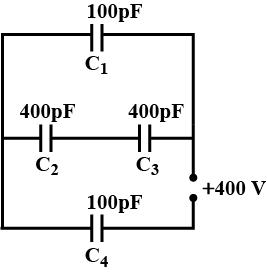• a)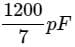• b)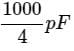• c)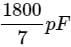• d)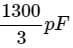### Related TestIas Masters Feb 12, 2022Capacitance of C1 = C4 = 100pF
Capacitance of C2 = C3 = 400pF
Supply voltage , V = 400V
Capacitors C2 and C3 are connected is series,
Equivalent capacitance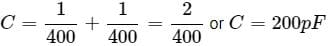Capacitors C1 and C are in parallel
Their equivalent capacitance.
C = C + C1 = 200 + 100 = 300pF
Capacitors C'' and C4 are connected in series Equivalent capacitance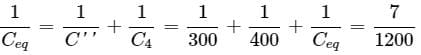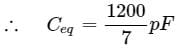Capacitance of C1 = C4 = 100pFCapacitance of C2 = C3 = 400pFSupply voltage , V = 400VCapacitors C2 and C3 are connected is series,Equivalent capacitanceCapacitors C1 and C are in parallelTheir equivalent capacitance.C = C + C1 = 200 + 100 = 300pFCapacitors C and C4 are connected in series Equivalent capacitance
Do you know? How Toppers prepare for NEET Exam
With help of the best NEET teachers & toppers, We have prepared a guide for student who are preparing for NEET : 15 Steps to clear NEET Exam